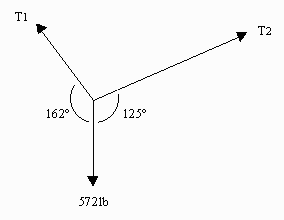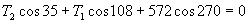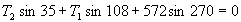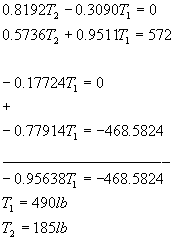# Occupational Health and Safety: Determining Tension in RopesFind the tensions T1 and T2 in the ropes as shown in the diagram to the right.

## Solution

Recall that in order for an object to be static the sum of the vertical forces on it must = 0 and the sum of the horizontal forces on it must = 0. This means that for the point where the three ropes join:

Horizontal forces:Vertical forces:If you apply the sin and cosine functions with your calculators you get two equations in two unknowns as shown. The first step is to eliminate one of the variables. In the solution shown below, Row 1 was multiplied by 0.5736 and Row 2 was multiplied by -0.8192 and then the two equations were added. Once T1 has been found, T2 can be found by substitution.Written by Louise Routledge, September 1, 1998